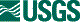## ISIS 2ISIS Application Documentation

# algebra

Printer Friendly View | TOC | Home

Performs algebra on either one or two cubes

 Overview Parameters

## Description

This program will perform simple algebra on either one or two cubes. The two cubes may be added, subtracted, multiplied or divided. The following equations are used:
```    UNARY:      out = (A * from1) + C
ADD:        out = ((from1 - D) * A) + ((from2 - E) * B) + C
SUBTRACT:   out = ((from1 - D) * A) - ((from2 - E) * B) + C
MULTIPLY:   out = ((from1 - D) * A) * ((from2 - E) * B) + C
DIVIDE:     out = ((from1 - D) * A) / ((from2 - E) * B) + C
```
The FROM2 cube must have either one band or the same number of bands as the FROM cube. If the FROM2 cube has one band, then the algebraic formula will be applied to all bands in FROM using that single band in FROM2. If FROM2 is a multi-band cube, the algebra will be performed between corresponding bands from FROM and FROM2.

## Related Applications to Previous Versions of ISIS

This program replaces the following applications existing in previous versions of ISIS:
• mult

## History

 Jim Mathews 1994-02-09 Original version Tracie Sucharski 2002-08-20 Ported to Isis 3.0, combining add,mad,b16mad and mult. Tracie Sucharski 2002-10-29 Was not handling special pixel values correctly. If only one input file, output the input special pixel value. If two input files and one of the input values is a special pixel value, output a NULL. Tracie Sucharski 2002-11-12 Changed parameters MULT1,MULT2 and ADD to A,B and C respectively. Tracie Sucharski 2003-01-28 Removed from Utility category. Stuart Sides 2003-05-16 Modified schema location from astogeology... to isis.astrogeology..." Stuart Sides 2003-07-29 Modified filename parameters to be cube parameters where necessary Stuart Sides 2003-10-23 Fixed typo in algebra.xml which caused IsisGui to display names and input fields incorrectly. Jeff Anderson 2004-02-17 Fixed division by zero problem with DIV operator and bug with single files by adding UNARY operator. Jeff Anderson 2004-02-17 Fixed division by zero problem with DIV operator and bug with single files by adding UNARY operator. Drew Davidson 2005-07-27 Added the D and E coefficients Kris Becker 2006-12-14 Modifed how special pixels are propagated to the output file. Originally, all special pixel values and operations resulted in Null being set to the output pixel. Now if the FROM pixel is a special pixel, it is always propagated to the output file unchanged without regard of the FROM2 pixel. If FROM is not a special pixel and FROM2 is, the output pixel is set to Null. Stacy Alley 2007-08-20 Fixed segmenation fault error. If the user did not enter a FROM2 value then tried to perform a non-unary operation the program would crash. FIX:The program will inform the user of the error without crashing so they can make the proper correction. Mackenzie Boyd 2011-04-11 Changed parameter FROM1 to be FROM Steven Lambright 2012-02-23 Updated to use the new ProcessByLine API. This program now takes advantage of multiple global processing threads.

## Parameter Groups

### Files

Name Description
FROM First Input cube
FROM2 Second Input Cube
TO Output cube

### Operators and Coefficients

Name Description
OPERATOR Operation performed on cubes
A Multiplicative constant for first input cube
B Multiplicative constant for second input cube

### Files: FROM

#### Description

Use this parameter to select the cube which is the first term in the equation.

 Type cube input *.cub

### Files: FROM2

#### Description

This image will be used as the second term in the equation. This cube must have either one band or the same number of bands as the cube in FROM. If this cube has one band, then the single band will be applied to each band in FROM using the equation. If this cube has multi-bands then each corresponding band in FROM2 is applied to FROM using the equation.

 Type cube input *.cub

### Files: TO

#### Description

This file will contain the results of the algebraically manipulated cubes.

 Type cube output real

### Operators and Coefficients: OPERATOR

#### Description

Mathematical operation performed on the two input cubes

Type string
Default SUBTRACT
Option List:
Option Brief Description
UNARYOperate on first input cube Apply the following equation: out = A * from1 + C

#### Exclusions

• FROM2
• B
• D
• E
ADDAdd images Add images together using following equation: out = ((from1 - D) * A) + ((from2 - E) * B) + C

#### Inclusions

• FROM2
SUBTRACTSubtract images Subtract FROM2 from FROM using the following equation: out = ((from1 - D) * A) - ((from2 - E) * B) + C

#### Inclusions

• FROM2
MULTIPLYMultiply images Multiply images together using the following equation: out = ((from1 - D) * A) * ((from2 - E) * B) + C

#### Inclusions

• FROM2
DIVIDEDivide images Divide FROM by FROM2 using the following equation: out = ((from1 - D) * A) + ((from2 - E) * B) + C

• FROM2

### Operators and Coefficients: A

#### Description

This defines the multiplicative constant for the first input cube.

 Type double 1.0

### Operators and Coefficients: B

#### Description

This defines the multiplicative constant for the second input cube.

 Type double 1.0

### Operators and Coefficients: C

#### Description

This defines the additive constant for entire equation.

 Type double 0.0

### Operators and Coefficients: D

#### Description

This defines the additive constant for the first input cube.

 Type double 0.0

### Operators and Coefficients: E

#### Description

This defines the additive constant for second input cube.

 Type double 0.0